Most Affordable JEE | NEET | 8,9,10 Preparation by Kota's Top IITian Doctor Faculties

# Mole Concept - JEE Main Previous Year Questions with SolutionsJEE Main Previous Year Papers Questions of Chemistry With Solutions are available at eSaral. Simulator Previous Years AIEEE/JEE Mains Questions
Q. A 5.2 molal aqueous solution of methyl alcohol, $\mathrm{CH}_{3} \mathrm{OH}$, is supplied. What is the mole fraction of methyl alcohol in the solution ? (1) 0.086           (2) 0.050             (3) 0.100             (4) 0.190 [AIEEE-2011]
Ans. (1) $5.2 \mathrm{m} \mathrm{CH}_{3} \mathrm{OH}$ ie $5.2 \mathrm{mol} \mathrm{CH}_{3} \mathrm{OH}$ present in $1 \mathrm{kg}$ water $\mathrm{X}_{\mathrm{M}}=\frac{5.2}{5.2+\frac{1000}{18}}=\frac{5.2}{5.2+55.5}=\frac{5.2}{60.7}=.086$
Q. The concentrated sulphuric acid that is peddled commercially is 95% $\mathrm{H}_{2} \mathrm{SO}_{4}$ by weight. If the density of this commerical acid is 1.834 g $\mathrm{cm}^{-3}$, the molarity of this solution is :- (1) 17.8 M         (2) 15.7 M           (3) 10.5 M           (4) 12.0 M [aieee-2012]
Ans. (1) $95 \% \frac{\mathrm{w}}{\mathrm{w}} \mathrm{H}_{2} \mathrm{SO}_{4}$ i.e. $100 \mathrm{gm}$ contain $95 \mathrm{gm} \mathrm{H}_{2} \mathrm{SO}_{4}$ Molarity $=\frac{95 / 98}{100 / 1.834} \times 1000$ $=\frac{95 \times 1.834}{98 \times 100} \times 1000=1.78$
Q. The density of a solution prepared by dissolving 120 g of urea (mol. mass = 60 u) in 1000 g of water is 1.15 g/mL. The molarity of this solution is (1) 2.05 M           (2) 0.50 M            (3) 1.78 M             (4) 1.02 M [AIEEE-2012]
Ans. (1) Mass of Solution = 120 + 1000 = 1120 gm Vol of solution $=\frac{\text { mass }}{\text { density }}$ $=\frac{1120}{1.15} \mathrm{ml}$ Molarity $=\frac{120 / 60}{1120 / 1.15}=\frac{2 \times 1.15}{1120} \times 1000=2.05 \mathrm{M}$
Q. A transition metal M forms a volatile chloride which has a vapour density of 94.8. If it contains 74.75% of chlorine the formula of the metal chloride will be (1) $\mathrm{MCl}_{2}$ (2) $\mathrm{MCl}_{4}$ (3) $\mathrm{MCl}_{5}$ (4) $\mathrm{MCl}_{3}$ [AIEEE 2012 (Online)]
Ans. (2) Mol. wt = 189.6 mass of $\mathrm{C} \ell=\frac{74.75}{100} \times 189.6=141.7 \mathrm{gm}$ formula: $\mathrm{MCl}_{4}$
Q. The ratio of number of oxygen atoms (O) in 16.0g ozone $\left(\mathrm{O}_{3}\right)$, 28.0 g carbon monoxide (CO) and 16.0g oxygen $\left(\mathrm{O}_{2}\right)$ is : (Atomic mass : $\mathrm{C}=12, \mathrm{O}=16$ and Avogadro's constant $\mathrm{N}_{\mathrm{A}}=6.0 \times 10^{23} \mathrm{mol}^{-1}$ ) (1) 3 : 1 : 1         (2) 1 : 1 : 2            (3) 3 : 1 : 2            (4) 1 : 1 : 1 [AIEEE 2012 (Online)]
Ans. (4) $\frac{16}{48} \times 3 \times \mathrm{N}_{\mathrm{A}}: \frac{28}{28} \times 1 \times \mathrm{N}_{\mathrm{A}}: \frac{16}{32} \times 2 \times \mathrm{N}_{\mathrm{A}}$ 1 : 1 : 1
Q. When $\mathrm{CO}_{2}$ (g) is passed over red hot coke it partially gets reduced to CO(g). Upon passing 0.5 litre of $\mathrm{CO}_{2}$ (g) over red hot coke, the total volume of the gases increased to 700 mL. The composition of the gaseous mixture at STP is :- (1) $\mathrm{CO}_{2}=200 \mathrm{mL} ; \mathrm{CO}=500 \mathrm{mL}$ (2) $\mathrm{CO}_{2}=350 \mathrm{mL} ; \mathrm{CO}=350 \mathrm{mL}$ (3) $\mathrm{CO}_{2}=0.0 \mathrm{mL} ; \mathrm{CO}=700 \mathrm{mL}$ (4) $\mathrm{CO}_{2}=300 \mathrm{mL} ; \mathrm{CO}=400 \mathrm{mL}$ [AIEEE 2012 (Online)]
Ans. (4)
Q. An open vessel at 300 K is heated till $\frac{2}{5}$ th of the air in it is expelled. Assuming that the volume of the vessel remains constant, the temperature to which the vessel is heated is :- (1) 750 K        (2) 400 K            (3) 500 K          (4) 1500K [AIEEE 2012 (Online)]
Ans. (3)
Q. The density of 3M solution of sodium chloride is 1.252 g $\mathrm{mL}^{-1}$. The molality of the solution will be (molar mass, NaCl = 58.5 g $\mathrm{mol}^{-1}$) (1) 2.18 m          (2) 3.00 m            (3) 2.60 m           (4) 2.79 m [JEE(Main-online)-2013]
Ans. (4) $\mathrm{m}=\frac{\mathrm{M} \times 1000}{1000 \mathrm{d}-\mathrm{MMw}}=\frac{3 \times 1000}{1000 \times 1.252-3 \times 58.5}$ $=\frac{3000}{1252-175.5}=\frac{3000}{1076.5}=2.79$
Q. 10 mL of 2(M) NaOH solution is added to 200 mL of 0.5 (M) of NaOH solution. What is the final concentration ? (1) 0.57 M          (2) 5.7 M              (3) 11.4 M            (4) 1.14 M [JEE(Main-online)-2013]
Ans. (1) $\mathrm{M}_{\mathrm{f}}=\frac{2 \times 10+0.5 \times 200}{210}$ $=\frac{20+100}{210}=\frac{120}{210}=.57$
Q. Number of atoms in the following samples of substances is the largest in : (1) 127.0g of iodine (2) 48.0g of magnesium (3) 71.0g of chlorine (4) 4.0g of hydrogen [JEE(Main) 2013 (Online)]
Ans. (4)
Q. A gaseous hydrocarbon gives upon combustion 0.72 g of water and 3.08 g of CO2. The empirical formula of the hydrocarbon is (1) $\mathrm{C}_{2} \mathrm{H}_{4}$ (2) $\mathrm{C}_{3} \mathrm{H}_{4}$ ( 3) $\mathrm{C}_{6} \mathrm{H}_{5}$ (4) $\mathrm{C}_{7} \mathrm{H}_{8}$ [JEE(Main)-2013]
Ans. (4) $\mathrm{C}_{\mathrm{x}} \mathrm{H}_{\mathrm{y}}+\left(\mathrm{x}+\frac{\mathrm{y}}{4}\right) \mathrm{O}_{2} \rightarrow \mathrm{x} \mathrm{CO}_{2}+\frac{\mathrm{y}}{2} \mathrm{H}_{2} \mathrm{O}$ a' moles $\quad$ xa' $\quad\left(\frac{\mathrm{y}}{2} \times \mathrm{a}\right)$ $\frac{\mathrm{x}}{\mathrm{y} / 2}=\frac{3.08 / 44}{0.72 / 18}=\mathrm{C}_{7} \mathrm{H}_{8}$
Q. For the estimation of nitrogen, 1.4 g of an organic compound was digested by Kjeldahl method and the evolved ammonia was absorbed in 60 mL of $\frac{\mathrm{M}}{10}$ sulphuric acid. The unreacted acid required 20 mL of $\frac{\mathrm{M}}{10}$ sodium hydroxide for complete neutralizaton. The percentage of nitrogen in the compound is : (1) 3% (2) 5% (3) 6% (4) 10% [JEE(Main-online)-2014]
Ans. (4)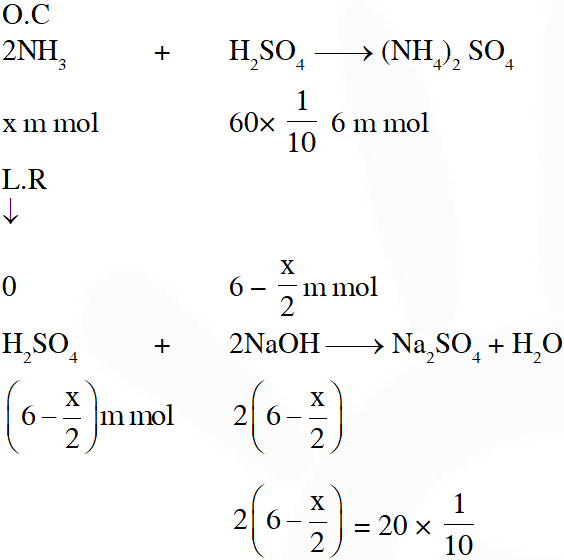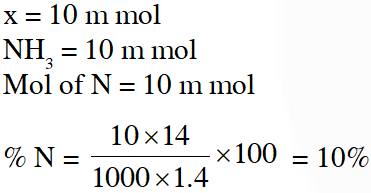Q. The amount of $\mathrm{BaSO}_{4}$ formed upon mixing 100 mL of 20.8% $\mathrm{BaCl}_{2}$ solution with 50 mL of 9.8% $\mathrm{H}_{2} \mathrm{SO}_{4}$ solution will be : (Ba = l37, Cl = 35.5, S=32, H = l and O = 16) (1) 33.2 g           (2) 11.65 g            (3) 23.3 g             (4) 30.6 g [JEE(Main-online)-2014]
Ans. (2)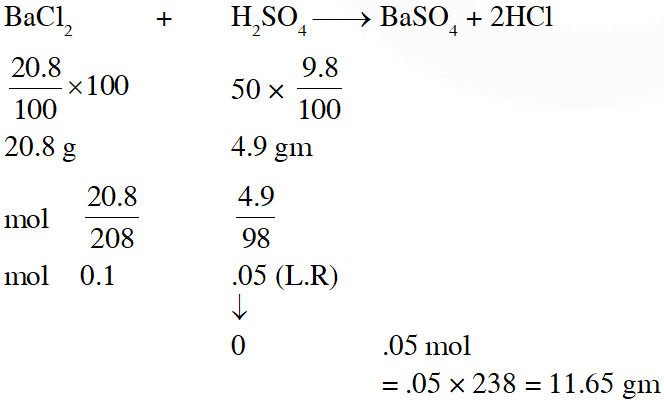Q. The ratio of masses of oxygen and nitrogen in a particular gaseous mixture is 1 : 4. The ratio of number of their molecule is : (1) 1 : 8         (2) 3 : 16           (3) 1 : 4          (4) 7 : 32 [JEE(Main)-2014]
Ans. (4) Given $\frac{\mathrm{W}_{\mathrm{O}_{2}}}{\mathrm{W}_{\mathrm{N}_{2}}}=\frac{1}{4} \Rightarrow \frac{\mathrm{n}_{\mathrm{O}_{2}}}{\mathrm{n}_{\mathrm{N}_{2}}}=\frac{\mathrm{W}_{\mathrm{O}_{2}} \times \mathrm{M}_{\mathrm{N}_{2}}}{\mathrm{W}_{\mathrm{N}_{2}} \times \mathrm{M}_{\mathrm{O}_{2}}}=\frac{1}{4} \times \frac{28}{32}=\frac{7}{32}$
Q. The molecular formula of a commercial resin used for exchanging ions in water softening is $\mathrm{C}_{8} \mathrm{H}_{7} \mathrm{SO}_{3} \mathrm{Na}$ (Mol. w.t 206). What would be the maximum uptake of $\mathrm{Ca}^{2+}$ ions by the resin when expressed in mole per gram resin ? (1) $\frac{2}{309}$         (2) $\frac{1}{412}$           (3) $\frac{1}{103}$             (4) $\frac{1}{206}$ [JEE(Main)-2015]
Ans. (2) For softening of hard water by ion exchange resin method, reaction involved is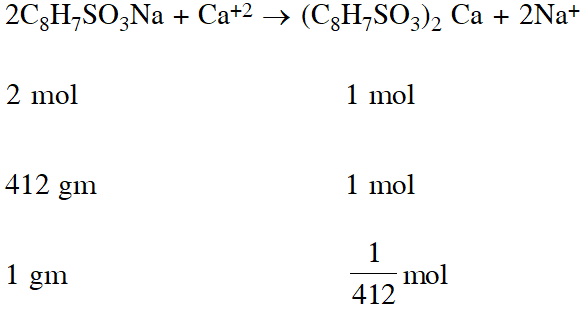Q. 3g of activated charcoal was added to 50 mL of acetic acid solution (0.06N) in a flask. After an hour it was filtered and the strength of the filtrate was found to be 0.042 N. The amount of acetic acid adsorbed (per gram of charcoal) is : (1) 42 mg         (2) 54 mg          (3) 18 mg          (4) 36 mg [JEE(Main)-2015]
Ans. (3) $\because$ Number of moles of $\mathrm{CH}_{3} \mathrm{COOH}$ adsorbed $=(0.06-0.042) \times \frac{50}{1000}$ $\therefore$ Amount of $\mathrm{CH}_{3} \mathrm{COOH}$ adsorbed per gram of charcoal $=\frac{0.018 \times 50}{1000} \times \frac{60}{3}=0.018 \mathrm{gm}$ = 18 mg
Q. In Carius method of estimation of halogens, 250 mg of an organic compound gave 141 mg of AgBr. The percentage of bromine in the compound is : (at. mass Ag = 108; Br = 80) (1) 48        (2) 60        (3) 24           (4) 36 [JEE(Main)-2015]
Ans. (3)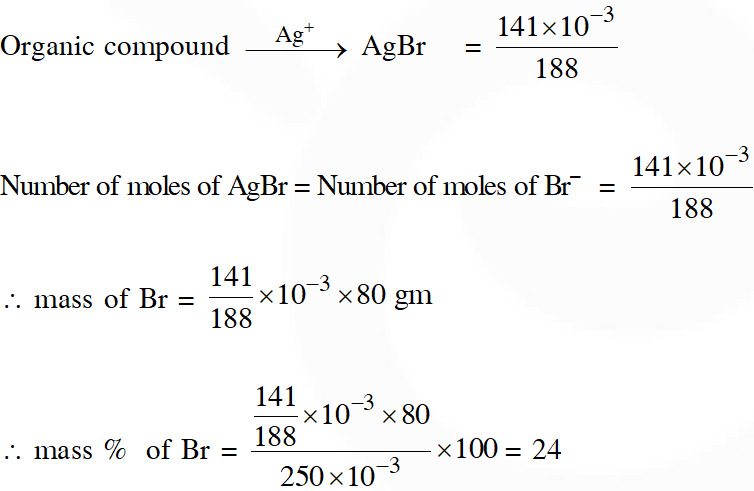Q. At 300 K and 1 atm, 15 mL of a gaseous hydrocarbon requires 375 mL air containing 20% $\mathrm{O}_{2}$ by volume for complete combustion. After combustion the gases occupy 330 mL. Assuming that the water formed is in liquid form and the volumes were measured at the same temperature and pressure, the formula of the hydrocarbon is :- (1) $\mathrm{C}_{4} \mathrm{H}_{10}$ (2) $\mathrm{C}_{3} \mathrm{H}_{6}$ (3) $\mathrm{C}_{3} \mathrm{H}_{8}$ (4) $\mathrm{C}_{4} \mathrm{H}_{8}$ [JEE(Main)-2016]
Ans. (3)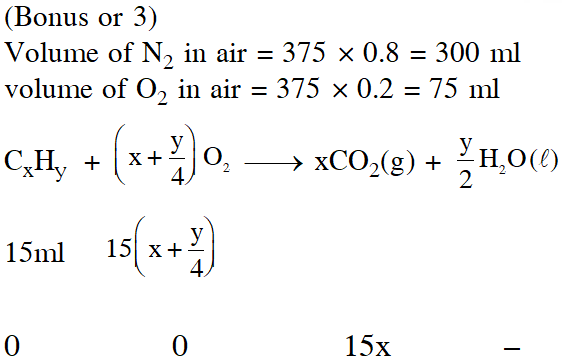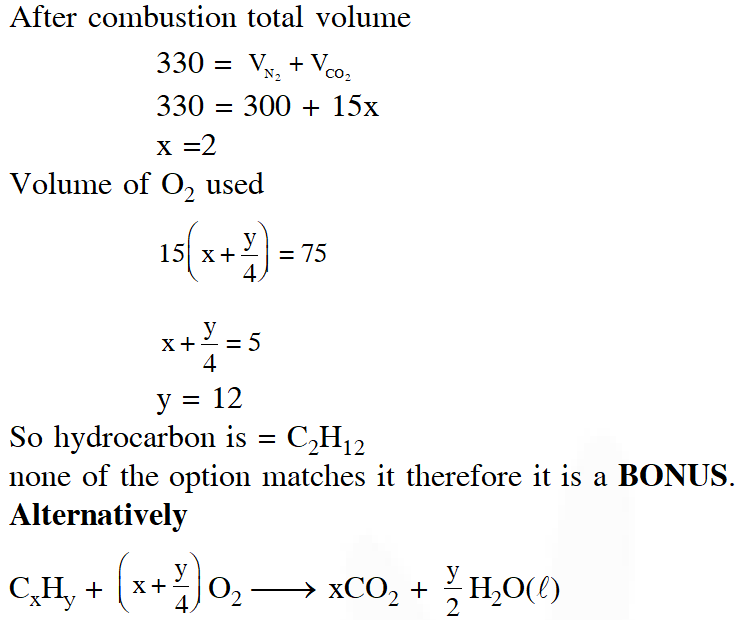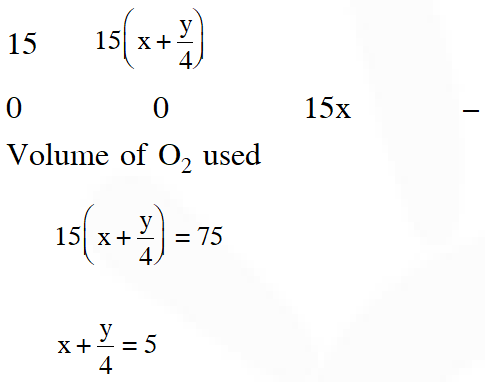If further information (i.e., 330 ml) is neglected, option (3) only satisfy the above equation.
Q. The concentration of fluoride, lead, nitrate and iron in a water sample from an underground lake was found to be 1000 ppb, 40 ppb, 100 ppm and 0.2 ppm, respectively. This water is unsuitable for drinking due to high concentration of :- (1) Iron             (2) Fluoride              (3) Lead             (4) Nitrate [JEE Main-2016]
Ans. (4)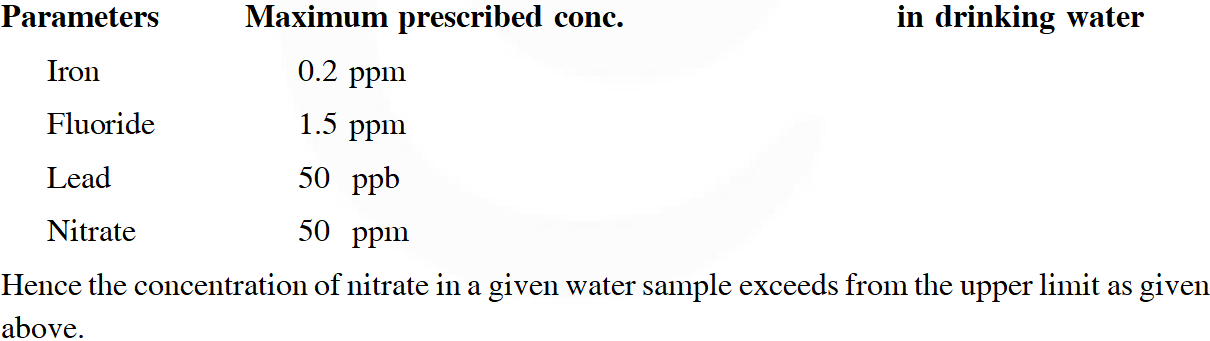Q. The most abundant elements by mass in the body of a healthy human adult are : Oxygen (61.4%) ; Carbon (22.9%), Hydrogen (10.0%) ; and Nitrogen (2.6%). The weight which a 75 kg person would gain if all 1H atoms are replaced by $^{2} \mathrm{H}$ atoms is (1) 15 kg             (2) 37.5 kg              (3) 7.5 kg              (4) 10 kg [JEE(Main)-2017]
Ans. (3) Mass in the body of a healthy human adult has :- Oxygen = 61.4%, Carbon = 22.9%, Hydrogen = 10.0% and Nitrogen = 2.6% Total weight of person = 75 kg Mass due to $1 \mathrm{H}$ is $=75 \times \frac{10}{100}=7.5 \mathrm{kg}$ $^{1} \mathrm{H}$ atoms are replaced by $^{2} \mathrm{H}$ atoms. So mass gain by person =7.5 kg
Q. 1 gram of a carbonate $\left(\mathrm{M}_{2} \mathrm{CO}_{3}\right)$ on treatment with excess HCl produces 0.01186 mole of $\mathrm{CO}_{2}$. the molar mass of $\mathrm{M}_{2} \mathrm{CO}_{3}$ in g $\mathrm{mol}^{-1}$ is :- (1) 1186            (2) 84.3             (3) 118.6             (4) 11.86 [JEE(Main)-2017]
Ans. (2) Given chemical eq $^{\mathrm{n}}$ $\mathrm{M}_{2} \mathrm{CO}_{3}+2 \mathrm{HCl} \longrightarrow 2 \mathrm{MCl}+\mathrm{H}_{2} \mathrm{O}+\mathrm{CO}_{2}$ 1gm 0.01186mol $\Rightarrow$ from the balanced chemical $\mathrm{eq}^{\mathrm{n}}$ $\frac{1}{\mathrm{M}}=0.01186$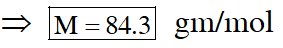.
Q. A water sample has ppm level concentration of following anions $\mathrm{F}^{-}=10 ; \mathrm{SO}_{4}^{2-}=100 ; \mathrm{NO}_{3}^{-}=50$ the anion/anions that make / makes the water sample unsuitable for drinking is / are : (1) only $\mathrm{NO}_{3}^{-}$ (2) both $\mathrm{SO}_{4}^{2-}$ and $\mathrm{NO}_{3}^{-}$ (3) only $\mathrm{F}^{-}$ (4) only $\mathrm{SO}_{4}^{2-}$ [JEE - Main 2017]
Ans. (3) $\mathrm{NO}_{3}^{-}$ : The maximum limit of nitrate in drinking water is 50 ppm. Excess nitrate in drinking water can cause disease. Such as methemoglobinemia. $\mathrm{SO}_{4}^{2-}:$ above 500 ppm of $\mathrm{SO}_{4}^{2-}$ ion in drinking water causes laxative effect otherwise at moderate levels it is harmless $\mathrm{F}^{-}$ : Above 2ppm concentration of $\mathrm{F}^{-}$ in drinking water cause brown mottling of teeth. The concentration given in question of $\mathrm{SO}_{4}^{2-} \& \mathrm{NO}_{3}^{-}$ in water is suitable for drinking but the concentration of $\mathrm{F}^{-}$ (i.e 10 ppm) make water unsuitable for drinking purp
Q. The ratio of mass percent of C and H of an organic compound $\left(\mathrm{C}_{\mathrm{x}} \mathrm{H}_{\mathrm{Y}} \mathrm{O}_{\mathrm{Z}}\right)$ is 6 : 1. If one molecule of the above compound $\left(\mathrm{C}_{\mathrm{x}} \mathrm{H}_{\mathrm{Y}} \mathrm{O}_{\mathrm{Z}}\right)$ contains half as much oxygen as required to burn one molecule of compound $\mathrm{C}_{\mathrm{X}} \mathrm{H}_{\mathrm{Y}}$ completely to $\mathrm{CO}_{2}$ and $\mathrm{H}_{2} \mathrm{O}$. The empirical formula of compound $\mathrm{C}_{\mathrm{X}} \mathrm{H}_{\mathrm{Y}} \mathrm{O}_{\mathrm{Z}}$ is : (1) $\mathrm{C}_{2} \mathrm{H}_{4} \mathrm{O}$ (2) $\mathrm{C}_{3} \mathrm{H}_{4} \mathrm{O}_{2}$ (3) $\mathrm{C}_{2} \mathrm{H}_{4} \mathrm{O}_{3}$ (4) $\mathrm{C}_{3} \mathrm{H}_{6} \mathrm{O}_{3}$ [JEE(Main)-2018]
Ans. (3)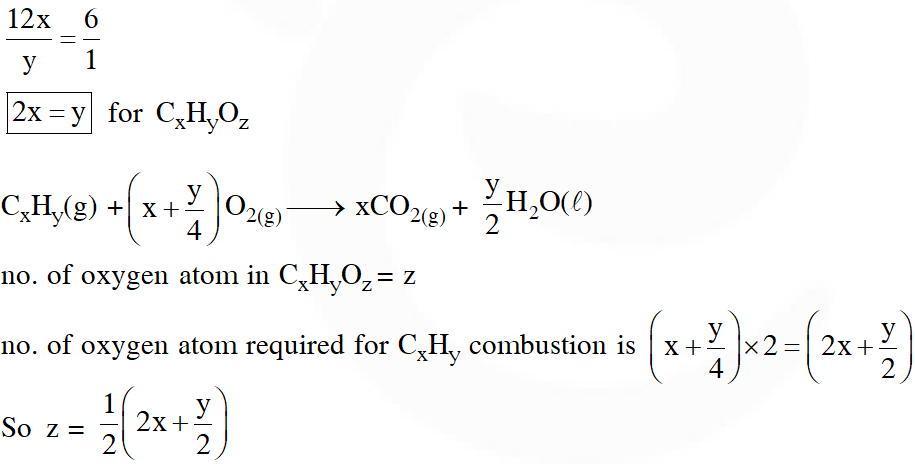Ashwamegh
Oct. 5, 2023, 6:35 a.m.
Thanks 🙏🙏👍👍
June 23, 2023, 6:35 a.m.
Sir Hindi medium me hai kya
Tejas Kesarwani
June 14, 2023, 6:35 a.m.
Nice questions for practice
‎ ‎
Nov. 15, 2022, 9:16 p.m.
🤔
Fearless
July 15, 2022, 5:38 p.m.
This question are easy
Hi
Feb. 8, 2022, 5:07 p.m.
Hi
Kaneriya smit
Dec. 22, 2021, 10:04 a.m.
Bohoti ache saval he or concept clear ho to assnise ho jayege question
Divyansh Shukla
Sept. 11, 2021, 1:17 p.m.
Nice prb sir ... 👍
Abhinav
Sept. 3, 2021, 12:02 p.m.
Everyone should try again this type of questions
Aug. 31, 2021, 8:16 p.m.
RK BRO IS ROCKING YOU KNOW
Aug. 31, 2021, 8:16 p.m.
RK BRO IS ROCKING
Nihal
Aug. 12, 2021, 12:27 p.m.
Thanks u really made my day
aman sharma
Aug. 12, 2021, 12:20 p.m.
really helpfull to make a strong grip in chemistry for jee mains
Im
June 12, 2021, 7:08 a.m.
thanks boss
Jiminsshi
May 22, 2021, 2:40 a.m.
6no sawal ka solution koi batao plz☹️
Neha rathod
April 29, 2021, 10:47 a.m.
thank you
hoon koi toh
April 17, 2021, 4:02 p.m.
maja aa gya
sejal saraf
Feb. 2, 2021, 11:55 a.m.
acha hai re
sejal saraf
Feb. 2, 2021, 11:54 a.m.
bc
N.G
Dec. 10, 2020, 5:12 p.m.
very ueful keep some more and more questions..thanku
pawan kalyan
Oct. 26, 2020, 12:36 p.m.
wtf
Sridhar
Oct. 21, 2020, 10:33 a.m.
When I click on solution it said that there is error.please solve that problem
Rithika Reddy
Oct. 15, 2020, 3:38 p.m.
Nice Awesome work👍
Anjali
Oct. 9, 2020, 11:58 p.m.
It's good 👍
Nanditha reddy
Oct. 5, 2020, 4:30 p.m.
Ujjwal Singh
Sept. 14, 2020, 10:07 a.m.
Thanks to give this type of questions
alok sharma class 11 iit jee aspirant
Sept. 2, 2020, 11:37 a.m.
sir pc me aapka aap kaise install kare
Aug. 17, 2020, 1:28 p.m.
thanks and good
Aug. 17, 2020, 1:28 p.m.
thanks and good after this information i have a AIR 621 in jee mains.
AYYAAN
Aug. 10, 2020, 6:54 p.m.
😊
July 26, 2020, 11:28 a.m.
very useful
Ankur towari
July 3, 2020, 8:01 p.m.
So good intiative hai sir
Shardul
June 24, 2020, 4:10 p.m.
Thanks a lot
king
June 2, 2020, 9:20 p.m.
good
divy prakash srivastav
May 25, 2020, 4:13 p.m.
please provide me daily tough questions {DPP} of IIT JEE {mains} and {advance} on my email ID ; [email protected]
Akshitha
May 18, 2020, 4:25 p.m.
It's a helpful site to JEE and NEET aspirants 🙏
Sehba
May 16, 2020, 4 p.m.
How many questions are asked in JEE mains 2020 from mole concept ??
Nikita Mehra
May 15, 2020, 7:55 p.m.
April 26, 2020, 9:08 a.m.
Thanks you Alot .
RIYA
April 21, 2020, 12:03 p.m.